## Fractions

A fraction, or fractional number, is used to represent a part of a whole. Fractions consist of two numbers: a numerator (which is above the line) and a denominator (which is below the line).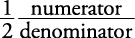The denominator tells you the number of equal parts into which something is divided. The numerator tells you how many of these equal parts are being considered. Thus, if the fraction is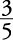of a pie, the denominator 5 tells you that the pie has been divided into five equal parts, of which 3 (numerator) are in the fraction. Sometimes, it helps to think of the dividing line (the middle of a fraction) as meaning “out of.” In other words,also means 3 out of 5 equal parts from the whole pie.

#### Negative fractions

Fractions may be negative as well as positive. (See the figure below.)

However, negative fractions are typically written as follows:#### Adding positive and negative fractions

The rules for signed numbers apply to fractions as well.

##### Example 1

1.2.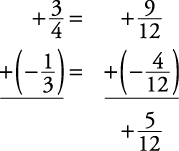#### Subtracting positive and negative fractions

The rule for subtracting signed numbers applies to fractions as well.

##### Example 2

Subtract the following.

1.2.3.#### Multiplying fractions

To multiply fractions, simply multiply the numerators and then multiply the denominators. Reduce to lowest terms if necessary.

##### Example 3

Multiply.This answer had to be reduced because it wasn't in lowest terms. Because whole numbers can also be written as fractions, and so forth, the problemwould be worked by changing 3 to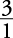.

#### Early reducing

Early reducing when multiplying fractions would have eliminated the need to reduce your answers after completing the multiplication. To reduce, find a number that divides evenly into one numerator and one denominator. In this case, 2 will divide evenly into the numerator 2 (it goes in one time) and into the denominator 12 (it goes in six times). Thus,Remember, you may only do early reducing when multiplying fractions. The rules for multiplying signed numbers hold here, too.

##### Example 4

Reduce early where possible and then multiply.

1.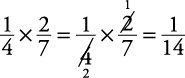2.#### Multiplying mixed numbers

To multiply mixed numbers, first change any mixed number to an improper fraction. Then multiply.

##### Example 5

Multiply.Change the answer, if in improper fraction form, back to a mixed number and reduce if necessary. Remember, the rules for multiplication of signed numbers apply here as well.

#### Dividing fractions

To divide fractions, invert (turn upside down) the second fraction (the one “divided by”) and multiply. Then reduce if possible.

##### Example 6

Divide.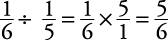Here, too, the rules for division of signed numbers apply.

#### Dividing complex fractions

Sometimes a division of fractions problem may appear in the following form (these are called complex fractions).

##### Example 7

Simplify.Consider the line separating the two fractions to mean “divided by.” Therefore, this problem can be rewritten as follows:Now, follow the same procedure as shown in Example .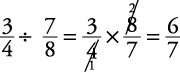#### Dividing mixed numbers

To divide mixed numbers, first change them to improper fractions. Then follow the rule for dividing fractions.

##### Example 8

Divide.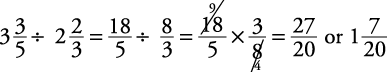Notice that after you invert and have a multiplication of fractions problem, you then may do early reducing when appropriate.